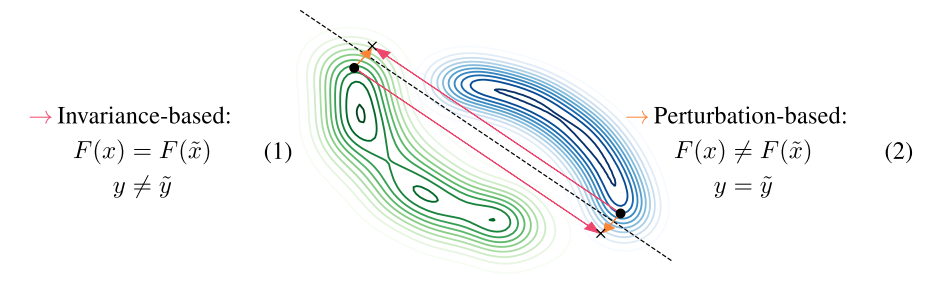# Excessive Invariance Causes Adversarial Vulnerability

Jacobsen et al., 2019Source: Jacobsen et al., 2019

## Summary

• Adversarial vulnerability in DNNs results from both sensitivity to task-irrelevant changes, as well as insensitivity to task-relevant changes
• Class-specific content can be manipulated without changing the hidden activations
• Identitfy standard cross-entropy loss as a reason and propose extension to the objective to encourage consideration of all task-relevant features
• Links: [ website ] [ pdf ]

## Background

• Adversarial examples demonstrate that while DNNs may demonstrate super-human performance on many tasks, tiny shifts to the input can cause them to make unintuitive mistakes
• Excessive invariance that causes adversarial vulnerability is likely a result of classifiers that rely only on a few highly predictive features
• Cross-entropy maximizes a bound on the mutual information between labels and representations, without incentivizing explaining all class-dependent variables

## Methods

• Complementary views of adversarial examples
• Perturbation-based: model produces a different output to the adversarial example, but the ground-truth (oracle) label is the same (nuisance perturbation)
• Invariance-based: model produces the same output to the adversarial example, but the ground-truth (oracle) label is different (semantic perturbation)
• Use fully invertible RevNet (bijective classifier) where first $C (< d)$ dimensions of $d$-dimensional representation is used as logits (semantic variables) and rest are unused (nuisance variables)
• Achieves comparable performance to popular CNNs (VGG19, ResNet-18)
• Create adversarial examples with metameric sampling: $x_{met} = F^{-1}(z_s, \tilde{z}_n)$, where $z_s$ and $\tilde{z}_n$ are from two different images
• Three ways to increase mutual information between label and bijective network representation $I(y;F_\theta(x))=I(y;z_s,z_n)=I(y;x)$:
• Directly increase $I(y;z_s)$
• Indirectly increase $I(y;z_s|z_n)$ by decreasing $I(y;z_n)$
• Reduce $I(z_s;z_n)$
• Independence cross-entropy loss for bijective networks adds term to minimize unused information $I(y;z_n)$ based on nuisance classifier

## Results

• Applying metameric sampling to fully invertible RevNet trained on MNIST and ImageNet shows that the nuisance variables dominate the visual appearance
• Same results when using feature adversaries on ResNet-152
• Using independence cross-entropy loss reduces invariance-based vulnerability compared to standard cross-entropy
• Results on shiftMNIST, where highly predictive features are introduced for training but removed in testing, shows a significant (but not total) reduction in error rate

## Conclusion

• The use of bijective networks to identify large subspaces of invariance-based vulnerability is interesting
• While the proposed independence cross-entropy loss had promising results, it is only applicable to bijective networks